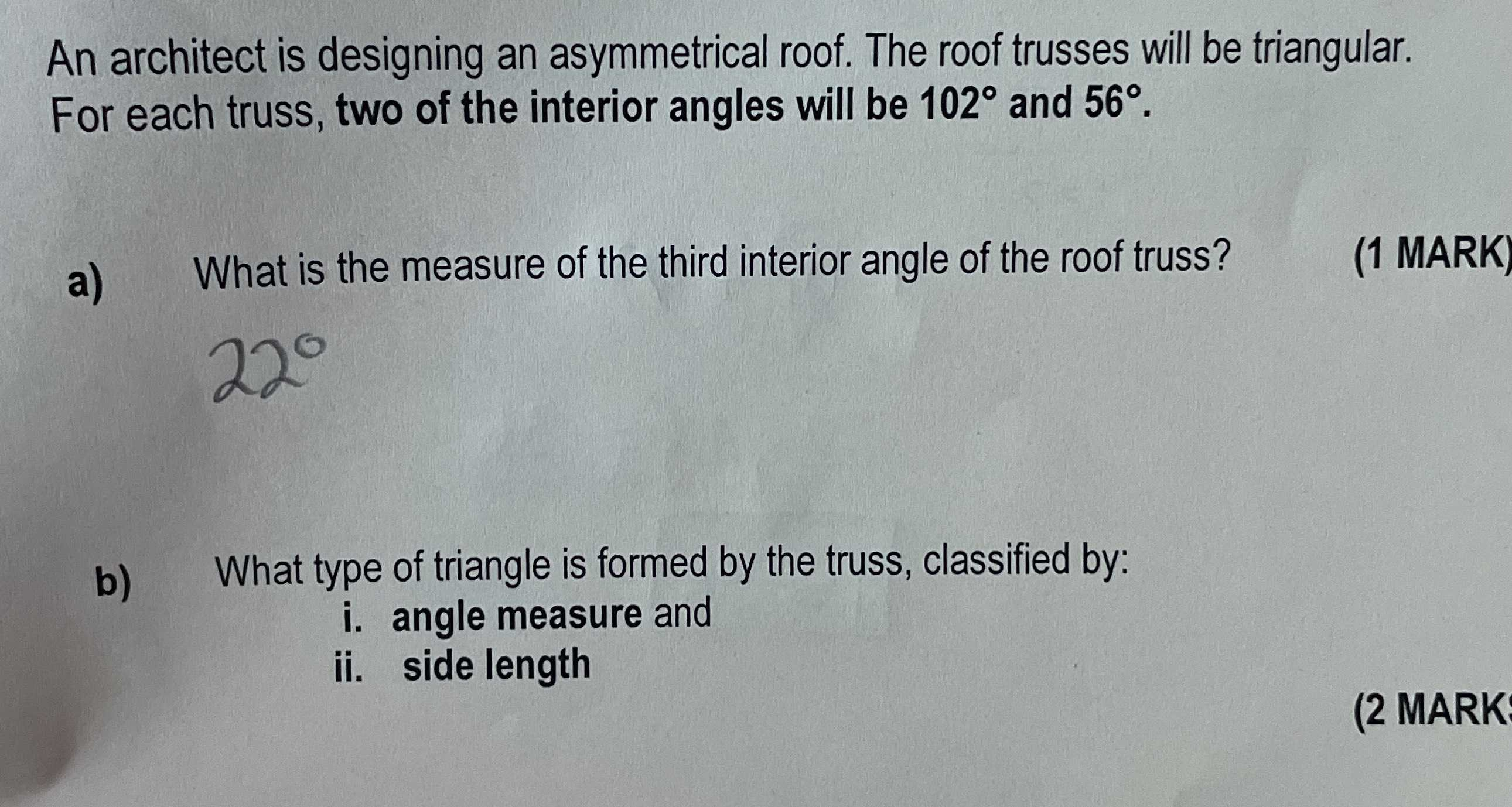### Still have math questions?

Algebra
QuestionAn architect is designing an asymmetrical roof. The roof trusses will be triangular. For each truss, two of the interior angles will be $$102 ^ { \circ }$$ and $$56 ^ { \circ }$$ . a) What is the measure of the third interior angle of the roof truss? (1 MARK) b) What type of triangle is formed by the truss, classified by: i. angle measure and ii. side length